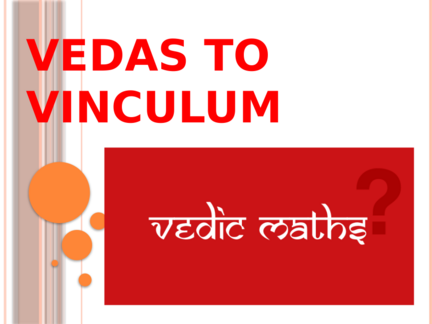# Anurupyena Sub Sutra– Multiplication in Vedic MathematicsContributed by:This PDF explain multiplication of numbers by anurupyena Sub Sutras and Vedic Mathematics.
1. VEDAS TO
2. JOURNEY THROUGH TEMPUS
Vedic Mathematics is
a book written by the Indian Hindu priest
jagadguru swami sri bharti krishna tirtha ji
(rediscovered from vedas between 1911 and
1918)
first published in 1965.
It contains a list of mental calulation
techniques based on the vedas.
The mental calculation system mentioned in
the book is also known by the same name or
as "Vedic Maths”
3. Vedicmathematics comprises “16
Sutras(formulae) & their
subsutras(corollaries)
At doer’s end:
doer has to identify and spot
certain characteristics , patterns
and then apply the sutra
( formula) which is applicable
there to
4. MEMENTOES
Reduces silly mistakes
Fastens the calculations
Intelligent guessing
Reduces burden
Makes mathematics a bit of
fun game for pupils
5. MULTIPLICATION BY 11 & ITS
MULTIPLES
(Sub sutra anurupyena)
Application of sutra is very
easy
Amazingly ; table of 11 is
not required
 Just have to add digits in
pairs
6. WORKING
2314× 11
Put single “0” in extreme left and
extreme right of the multiplicand
023140
(Single naught sandwich)
Add the digits in pair starting from
right to left
7. 023140
02314+0 (4+0=4)
0231+40 (1+4=5)
023+140 (3+1=4)
02+3140 (2+3=5)
0+23140 (0+2=2)
2314×11 = 25454
8. 0 2314 0
˟11
2+0 3+2 1+3 4+1 0+4
25454
9. IF SUM OF 2 DIGITS OF
MULTIPLICAND EXCEEDS “9”
When the sum exceeds 9 then carry the tens place
digit & add to the preceding digit
2824×11
Single naught sandwich=028240
0+4=4
4+2=6
2+8=10=10
8+2=10=10+1=11=11
2+0=2=2+1=3
Product=31064
10. MULTIPLICATION BY MULTIPLES
OF 11 (22,33,44.55.66…)
 Multiply the multiplicand by the
number of rank of the multiple
(e.g. 44 is 4th and 99 is 9th multiple so
multiply the multiplicand by 4 or 9 in
case we are multiplying by 44 or 99)
 Then apply the rule of multiplication
by 11 to the product obtained (single
naught sandwich)
11. 245
×22 (2×11)
245×2= 490
04900
51390
12. MULTIPLICATION BY 111
3496
×111
(Add 2 zeroes to the extreme right and
extreme left of the multiplicand)
Double Naught Sandwich
00 3496 00
Sandwiched Number=00349600
13. 3496
×111
00349600
(Double naught sandwich)
Add 3 digits at a time
starting from right
to left
14. 3 8 1 8 0
2 5
1 6
388056
15. MULTIPLICATION BY MULTIPLES
OF 111
(222 TO 999)
 Find the rank of the multiple
(for 555 the rank is 5, for 777 the
rank is 7 and so on)
 Multiply the multiplicand by the
number of rank
 Apply the multiplication of 111 the
product obtained
(Double Naught Sandwich
Method)
16. WORKING
1348
(2 × 111) × 222
(2 ×1348) 00 2696 00
00269600
(Double Naught Sandwich)
17. 00269600
2+0+0 6+2+0 9+6+2 0+0+6
6+9+6 0+6+9
6
8 17
21
5
8+1 17+2 21+1
9 19 22 1
299256
18. 19
sandwich the multiplicand
between single zero
 Multiply each digit of the
multiplicand by first digit of the
multiplier from right to left and
add to the immediate right digit
following it one by one
19. WORKING
0 1235 0
× 12
(2 ×0)+1=1 (2 ×2)+3=7 (2 ×3)+5=11 (2 ×5)+0=10
(2 ×1)+2=4
7+1 11+1
1 4 8 12 10
14820
20. SAME METHOD FOR 13 TO
19….
Practice the method
for
2356 ×15
2123 ×16
21. MULTIPLICATION OF SPECIAL NUMBERS
“Antyayordasake’pi”
Sum of unit digits are ten & rest
place digits are same
67
×63
 Sum of ten’s place digits is ten
& unit digits are same
22
×82
22. WORKING 67 ×63
 Multiply the unit digit & write the product in two digits
on one’s & ten’s place
 Multiply the ten’s place digit with its successor
67
×63
(7 ×3=21)
(6 × 7=42)
4221
23. 22
× 82
 Multiply the unit digits & write the product in two digits,
one’s & ten’s place
 Multiply the ten’s place digits & add one “unit digit” then
put in hundred's & thousand’s place
22
× 82
(2 ×2=04)
[(8 ×2)+2]
1804
24. PRACTICE THE METHOD..
58
× 52
75
× 35
25.  Gaurav Raj (TGT N/M)
GHS Dakahal Edu. Block: Kotkhai
Distt. Shimla
 Yogesh Kumar (TGT N/M)
GHS Samleu Edu. Block Banikhet
Distt. Chamba
 Sunita Bindra (TGT N/M)
GSSS Tutu Distt Shimla
26.